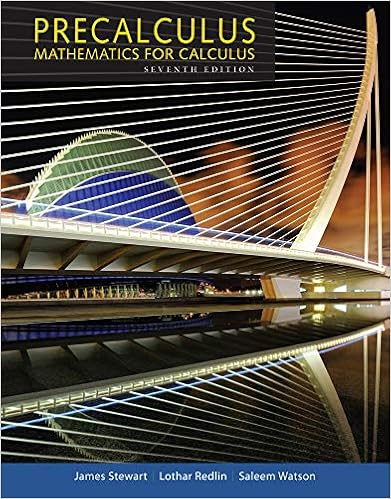# Whose coefficients are polynomials in s moreover if

• 72
• 100% (3) 3 out of 3 people found this document helpful

This preview shows page 30 - 32 out of 72 pages.

##### We have textbook solutions for you!
The document you are viewing contains questions related to this textbook.The document you are viewing contains questions related to this textbook.
Chapter 1 / Exercise 38
Precalculus: Mathematics for Calculus
Redlin/StewartExpert Verified
whose coefficients are polynomials in s. Moreover, if the coefficients of the given equation are polynomials of degree 1 in t , then (regardless of the order of the given equa- tion) the differential equation for is just a linear first-order equation. Since we know how to solve this first-order equation, the only serious obstacle we may encounter is obtaining the inverse Laplace transform of [This problem may be insurmountable, since the solution may not have a Laplace transform.] In illustrating the technique, we make use of the following fact. If is piecewise continuous on and of exponential order, then (12) (You may have already guessed this from the entries in Table 7.1, page 359.) An outline of the proof of (12) is given in Exercises 7.3, Problem 26. Solve the initial value problem (13) Let and take the Laplace transform of both sides of the equation in (13): (14) Using the initial conditions, we find E y F A s B s 2 Y A s B sy A 0 B y ¿ A 0 B s 2 Y A s B E y F A s B 2 E ty ¿ A t B F A s B 4 Y A s B 1 s . Y A s B E y F A s B y 2 ty ¿ 4 y 1 , y A 0 B y ¿ A 0 B 0 . lim s S E f F A s B 0 . 3 0, q B f A t B y A t B Y A s B . Y A s B Y A s B y A t B s 2 Y ¿ A s B 2 sY A s B y A 0 B . d ds 3 s 2 Y A s B sy A 0 B y ¿ A 0 B 4 E ty A t B F A s B d ds E y F A s B f A t B y A t B , n 1 d ds 3 sY A s B y A 0 B 4 sY ¿ A s B Y A s B . E ty ¿ A t B F A s B d ds E y ¿ F A s B f A t B y ¿ A t B , n 1 E t n f A t B F A s B A 1 B n d n F ds n A s B . 3 e t p cos 2 t 4 e t p sin 2 t e p t . w A t B y A t p B 3 e t p cos 3 2 A t p B 4 4 e t p sin 3 2 A t p B 4 e A t p B t p w A t B y A t p B . w A t p B y A t B , Section 7.5 Solving Initial Value Problems 379 Example 4 Solution
##### We have textbook solutions for you!
The document you are viewing contains questions related to this textbook.The document you are viewing contains questions related to this textbook.
Chapter 1 / Exercise 38
Precalculus: Mathematics for Calculus
Redlin/StewartExpert Verified
and Substituting these expressions into (14) gives (15) Equation (15) is a linear first-order equation and has the integrating factor (see Section 2.3). Multiplying (15) by we obtain Integrating and solving for yields (16) Now if is the Laplace transform of a piecewise continuous function of exponential order, then it follows from equation (12) that For this to occur, the constant C in equation (16) must be zero. Hence, and taking the inverse transform gives We can easily verify that is the solution to the given initial value problem by substituting it into (13). We end this section with an application from control theory. Let’s consider a servomech- anism that models an automatic pilot. Such a mechanism applies a torque to the steering control shaft so that a plane or boat will follow a prescribed course. If we let be the true direction (angle) of the craft at time t and be the desired direction at time t , then denotes the error or deviation between the desired direction and the true direction. Let’s assume that the servomechanism can measure the error and feed back to the steering shaft a component of torque that is proportional to but opposite in sign (see Figure 7.6 on page 381). Newton’s second law, expressed in terms of torques, states that A moment of inertia B A angular acceleration B total torque.
•••